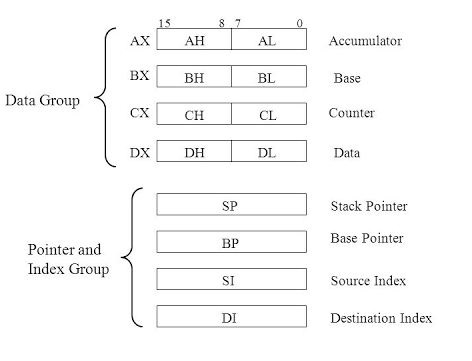# What are the CPU general purpose registers?

General purpose registers are additional registers that are present in CPU which is used for either memory address or data whenever needed. For example, storing current register content when there is an interruption.

### 8086 processor

Let us consider an 8086 processor. There are eight general purpose register in 8086 microprocessor which are explained below −### AX

This is the accumulator of 16 bits and is separated into two 8-bits registers AH and AL to likewise perform 8-bits instruction. It is usually utilized for arithmetical and logical directions, yet in 8086 chips it isn't required to have an accumulator as the objective operand.

Example − ADD AX, AX (AX= AX+AX)

### BX

This is the base register of 16 bits and is separated into two 8-bits registers BH and BL to likewise perform 8-bits instruction. It stores the value of offset.

Example − MOV BL,  (BL= 500H)

### CX

This is the counter register of 16 bits and is separated into two 8-bits registers CH and CL to likewise perform 8-bits instruction. It is generally utilized for looping and rotations.

Example

MOV CX,0005

MOV CL,5000

### DX

This is the data register of 16 bits and is separated into two 8-bits registers DH and DL to likewise perform 8-bits instruction. It is generally used for multiplication of an input/output port address.

Example − Mul BX (DX,AX=AX*BX)

### SP

This is the stack pointer of 16 bits that points to the topmost element of the stack.

### BP

This is the base pointer of 16 bits that is used to access parameters that have been passed in the stack.

### SI

This is the source index register of 16 bits that is used in pointer addressing of the data and as a source in string related operations.

### DI

This is the destination index register of 16 bits that is used in pointer addressing of the data and as a destination in string related operations.

### How to use general-purpose registers?

The 8 general-purpose registers of CPU that are capable of storing 32-digit binary numbers. In addition to 32-bit data, they can also store 16- or 8-bit data.

• When 32-bit data is stored, the instructions are represented as −

ER0, ER1, ER2, ER3, ER4, ER5, ER6, ER7

• When 16-bit data is stored, the instructions are represented using registers as 16 units −

E0, E1, E2, E3, E4, E5, E6, E7, R0, R1, R2, R3, R4, R5, R6, R7

• When 8-bit data is stored, the instructions are represented, using registers as 16 units −

R0H, R0L, R1H, R1L, R2H, R2L, R3H, R3L, R4H, R4L, R5H, R5L, R6H, R6L, R7H, R7L